Next: Nonlinear systems and chaos Up: Theory Previous: Dynamical systems   Contents

## Linear systems

Let us assume that the mapping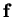in Equation (2.1) is linear, i.e.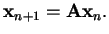(2.4)

Iterating the system for a given initial vectorleads to a sequence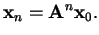(2.5)

The possible courses of evolution of such sequences can be characterized by the eigenvalues of the matrix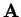. If there are eigenvalues that are greater than one in absolute value, almost all of the orbits will diverge to infinity. If all the eigenvalues are less than one in absolute value, the orbits will rapidly converge to the origin. Complex eigenvalues with absolute value of unity will lead to closed circular or elliptic orbits .

An affine map with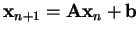behaves in essentially the same way. This shows that the autonomous linear system is too simple to describe any interesting dynamical phenomena, because in practice the only stable linear systems converge exponentially to a constant value.Next: Nonlinear systems and chaos Up: Theory Previous: Dynamical systems   Contents
Antti Honkela 2001-05-30